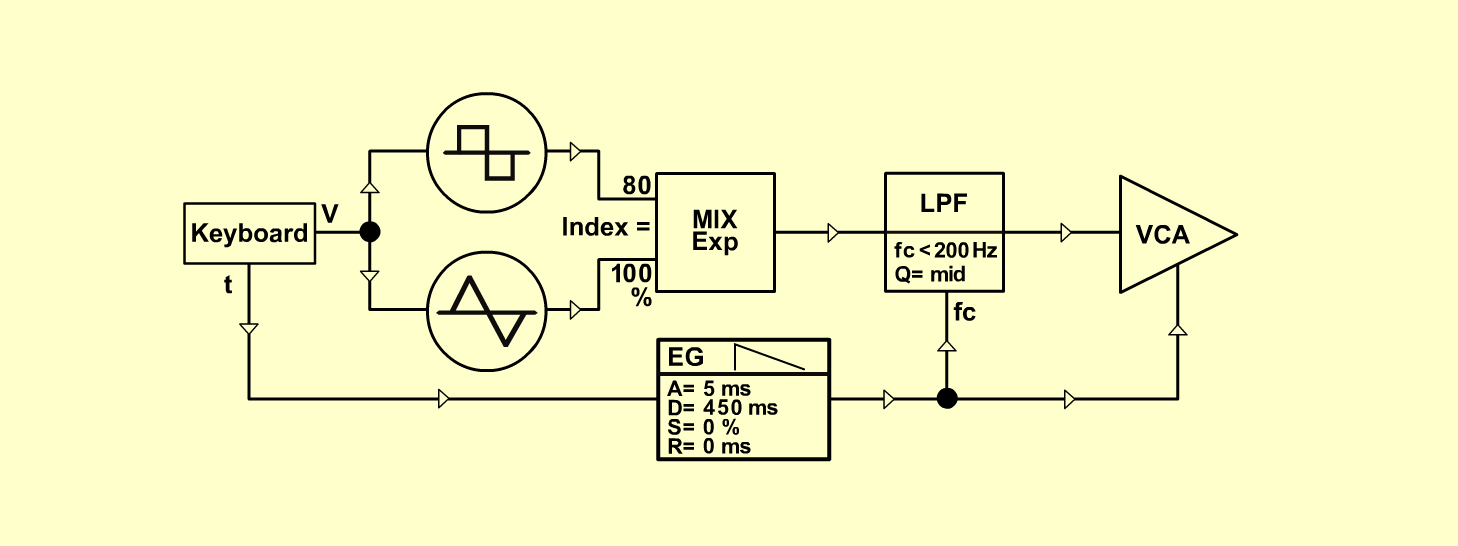Dry-BassInstrumentsD o e p f e r A - 100 Connections: Settings: A-110 (Square)  <=>  A-138 (Input 1)   A-110 (Tri)  <=>  A-138 (Input 2)   A-138 (Output)  <=>  A-120 (Audio In)   A-140 (Output/1)  <=>  A-120 (CV 1)   A-140 (Output/2)  <=>  A-131 (CV 1)   A-120 (Audio Out)  <=>  A-131 (Audio In)   A-131 (Audio Out)  <=> Amplifier   Pitch, Trigger and Gate: KB A-110 (PW = 5)   A-138 (In 1 = 8, In 2 = 10, Out = 10)   A-120 (Freq = 2, Lev = 10, Res = 5)   A-140 (A = 0.5, D = 5, S = 2, R = 5, Range = M)   A-131 (Gain = 0, Audio In 1 = 10,  Audio Out = 10) Josef MuellerSound sample Dry-Bass (65 Hz)